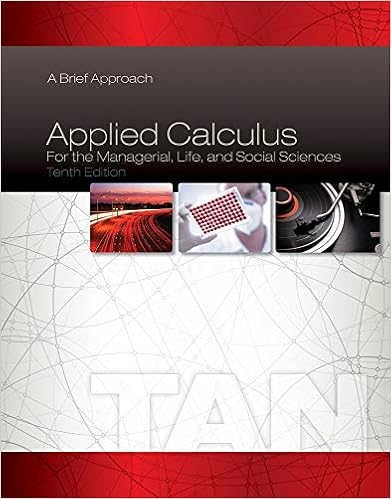# In the figure from chapter 3 trade for the home

• Test Prep
• 5
• 100% (7) 7 out of 7 people found this document helpful

This preview shows page 2 - 5 out of 5 pages.

##### We have textbook solutions for you!
The document you are viewing contains questions related to this textbook.The document you are viewing contains questions related to this textbook.
Chapter 2 / Exercise 48
Applied Calculus for the Managerial, Life, and Social Sciences: A Brief Approach
TanExpert Verified
8) In the figure (from chapter 3), trade for the Home country is: a. X M = 15, M A = 35; b. X M = 20, M A = 15; c. X M = 5, M A = 20; d. X M = 35, M A = 20; e. X M = 35, M A = 15;
##### We have textbook solutions for you!
The document you are viewing contains questions related to this textbook.The document you are viewing contains questions related to this textbook.
Chapter 2 / Exercise 48
Applied Calculus for the Managerial, Life, and Social Sciences: A Brief Approach
TanExpert Verified
3 Part 2: Questions. Provide detailed and clear answers. Clearly label axes and curves on each graph. 1) Ricardian model: Import demand curve: In the Ricardian model, consider Thailand and Germany. Thailand exports electronics and imports cars. Thailand’s production of electronics is Q E =100 under full specialization. Moreover, we assume that the relative price of cars is 1 in Autarky and we also assume that Thai consumers have Cobb-Douglas preferences in cars and electronics: a) Depending on the price of electronics, P E , what is the total GDP of Thailand? b) Depending on the relative price of cars P C /P E , how many cars does Thailand import? c) Draw Thailand’s import demand curve for cars. Also draw a hypothetical vertical export supply curve for cars for Germany (no calculation required for the export supply curve). d) Now, suppose that Germany welcomes many new immigrants, such that its size increases. What is the effect on the relative price of cars? (illustrate on previous graph) 2 / 1 2 / 1 E C Q Q U
4
•••